# What are Vector Diagrams? - Definition and Uses

An error occurred trying to load this video.

Try refreshing the page, or contact customer support.

Coming up next: Using Position vs. Time Graphs to Describe Motion

### You're on a roll. Keep up the good work!

Replay
Your next lesson will play in 10 seconds
• 0:00 What Are Vector Diagrams?
• 1:35 Subtracting Vectors
• 2:10 Examples
Save Save

Want to watch this again later?

Timeline
Autoplay
Autoplay
Speed Speed Audio mode

#### Recommended Lessons and Courses for You

Lesson Transcript
Instructor: David Wood

David has taught Honors Physics, AP Physics, IB Physics and general science courses. He has a Masters in Education, and a Bachelors in Physics.

After watching this video, you will be able to explain what vector diagrams are and how they are used, including vector addition and subtraction. A short quiz will follow.

## What Are Vector Diagrams?

Vector diagrams are simply diagrams that contain vectors. That's probably pretty obvious. But a more interesting question is to ask how they're used. A vector is an arrow that represents a quantity with both magnitude and direction. The length of the arrow represents the magnitude (or size) of the quantity, and the direction of the arrow represents the direction.

How do we use them? Well, you might have a vector diagram that shows the magnetic field created by a bar magnet, which looks like this: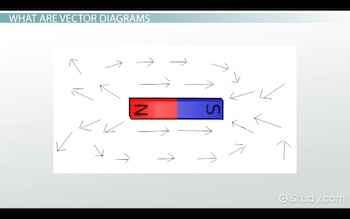Or you might have a vector diagram that shows the velocity of a projectile in the x and y direction during its flight, which looks like this: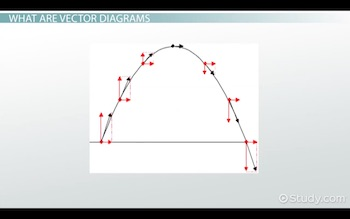But we can also use vector diagrams when we need to add or subtract vector quantities. And we're going to talk about that in more detail in this lesson.

When adding two vector quantities, you draw a vector arrow for each quantity, then you move one of them so that they're connected tip to tail. This means that the tip of one arrow is connected to the tail of the other arrow. Then draw a final arrow from the very start of the chain of vectors to the very end. This is your final result from adding the two vectors, which is why it's often known as a resultant.

So, this diagram shows vector A, added to vector B, to give a total, a resultant, represented by vector C: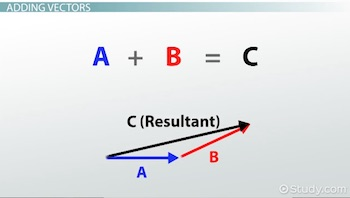If you were given a scale for the diagram, such as two centimeters equals three newtons of force, for example, you could then measure your result and find the number of newtons that this result represents. For example, you might need to do this for a question where you're finding the net force.

## Subtracting Vectors

Subtracting vectors is very similar. To subtract vectors, you take your two vectors, and then reverse the one you're subtracting. If this represents vector A, then negative vector A (or minus vector A) looks like this: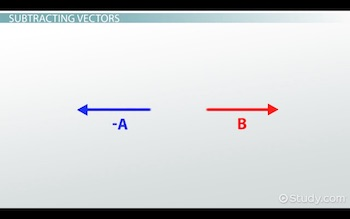Then, once you've reversed the vector you're subtracting, put them tip to tail in the same way you did when adding vectors. Draw a final arrow from the very start of the chain of vectors to the very end. This is your result from subtracting the two vectors.

So, this diagram shows vector A, subtracted from vector B, to give a final result, represented by vector C: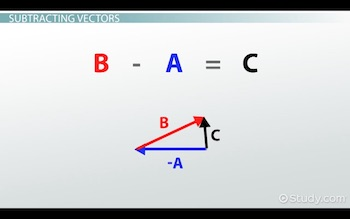## Example

Let's say you have these two vectors: vector A and vector B.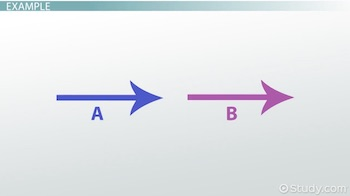And you're asked to find A plus B and also asked to find A minus B.

To find A plus B, you just put them tip to tail, like this: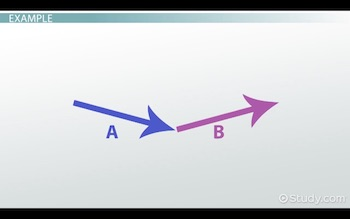Then draw an arrow from the start to the end. And that is your final result.

To find A minus B, we have to reverse the direction of B, like this: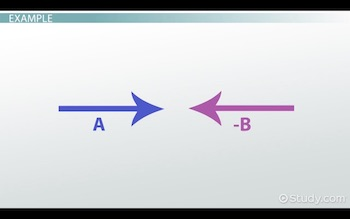Then put them tip to tail again. Finally, draw an arrow from the start to the end.

To unlock this lesson you must be a Study.com Member.

### Register to view this lesson

Are you a student or a teacher?

#### See for yourself why 30 million people use Study.com

##### Become a Study.com member and start learning now.
Back
What teachers are saying about Study.com

### Earning College Credit

Did you know… We have over 200 college courses that prepare you to earn credit by exam that is accepted by over 1,500 colleges and universities. You can test out of the first two years of college and save thousands off your degree. Anyone can earn credit-by-exam regardless of age or education level.